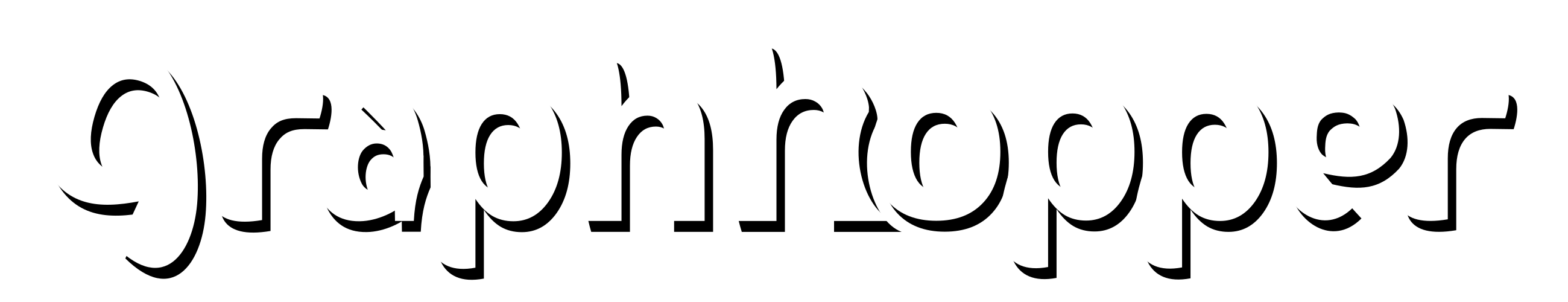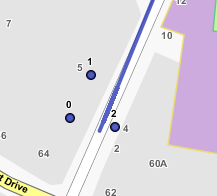# Low level api - odd path results

Hiyas, unsure where to post this as it is a little mix of everything.
My low level API is shown in the following post;

I can build a distance matrix but the path figures don’t seem to be correct.
As an example (references to map below);
From 3 -> To 2 - Distance = 0.0 - Time = 0.0
From 3 -> To 3 - Distance = 0.0 - Time = 0.0

From 3 -> To 7 - Distance = 0.0 - Time = 0.0
From 7 -> To 2 - Distance = 0.0 - Time = 0.0
From 7 -> To 3 - Distance = 0.0 - Time = 0.0

I would expect there to be some time and distance between these points.
Is there something I need to include or do differently?

``````private static BidirRoutingAlgorithm createAlgo(GraphHopperStorage graph) {
RoutingCHGraph routingCHGraph = graph.getRoutingCHGraph("rural");
CHRoutingAlgorithmFactory algoFactory = new CHRoutingAlgorithmFactory(routingCHGraph);
return algoFactory.createAlgo(new PMap().putObject(ALGORITHM, DIJKSTRA_BI));
}
``````

Once loaded into JSprit I get the following;
It is currently routing 0, 11, 10, 12, 1, 5, 2, 7, 3
I would expect it to be 0, 11, 10, 3, 2, 7, 12, 1, 5

Apologies for the poor line drawing.
Is it caused by the points being on the same edge?

As a temp work around (which I don’t really want to use long term) I have made a check to say if distance = 0 then use GraphHopper instead of Low lvl API

``````// path.getDistance() is the result from the low lvl api

if(path.getDistance() == 0 && (i != y)){
useGraphhopper(points, i, y);
}else{
matrix.setDistanceMeters(i, y, path.getDistance());
matrix.setTimeMilliseconds(i, y, path.getTime());
}
``````

The results using the above examples are;
From 3 -> To 2 -D- 22.013845143016813 -T- 1761.0
From 3 -> To 3 -D- 0.0 -T- 0.0

From 3 -> To 7 - Distance = 77.04845220778397 - Time = 6163.0
From 7 -> To 2 - Distance = 55.03460706476572 - Time = 4402.0
From 7 -> To 3 - Distance = 77.04845220778397 - Time = 6163.0

Edit

Something is wrong with my low lvl apiWhere 0 to 1 is low lvl api
0 -> 2 is graphhopper

To From Distance Time
0 0 0 0
0 1 938.622 75086
0 2 4.284524754 342